Download Presentation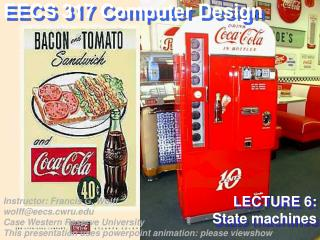LECTURE 6: State machines

# LECTURE 6: State machines - PowerPoint PPT Presentation

EECS 317 Computer Design. LECTURE 6: State machines. Instructor: Francis G. Wolff wolff@eecs.cwru.edu Case Western Reserve University This presentation uses powerpoint animation: please viewshow. Gated-Clock SR Flip-Flop (Latch Enable). PS. CLR. Q <= ( S NAND LE ) NAND NQ ;. S.I am the owner, or an agent authorized to act on behalf of the owner, of the copyrighted work described.
Download Presentation## LECTURE 6: State machines

An Image/Link below is provided (as is) to download presentation

Download Policy: Content on the Website is provided to you AS IS for your information and personal use and may not be sold / licensed / shared on other websites without getting consent from its author.While downloading, if for some reason you are not able to download a presentation, the publisher may have deleted the file from their server.

- - - - - - - - - - - - - - - - - - - - - - - - - - E N D - - - - - - - - - - - - - - - - - - - - - - - - - -
Presentation TranscriptEECS 317 Computer Design

LECTURE 6: State machines

Instructor: Francis G. Wolffwolff@eecs.cwru.eduCase Western Reserve University This presentation uses powerpoint animation: please viewshow

CWRU EECS 317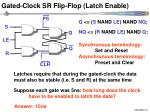Gated-Clock SR Flip-Flop (Latch Enable)

PS

CLR

Q <= (S NAND LE) NAND NQ;

S

NQ <= (R NAND LE) NAND Q;

Q

LE

Synchronous terminology: Set and Reset

Q

R

Asynchronous terminology: Preset and Clear

Latches require that during the gated-clock the data must also be stable (i.e. S and R) at the same time

Suppose each gate was 5ns: how long does the clock have to be enabled to latch the data?

CWRU EECS 317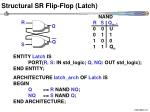Structural SR Flip-Flop (Latch)

NANDRSQn+10 0 U0 1 11 0 01 1 Qn

R

Q

Q

S

ENTITY Latch ISPORT(R, S: IN std_logic; Q, NQ: OUT std_logic);END ENTITY;

ARCHITECTURE latch_arch OF Latch ISBEGIN Q <= R NAND NQ;NQ <= S NAND Q;END ARCHITECTURE;

CWRU EECS 317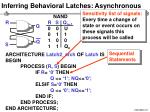Inferring Behavioral Latches: Asynchronous

Sensitivity list of signals:Every time a change of state or event occurs on these signals this process will be called

NANDRSQn+10 0 U0 1 11 0 01 1 Qn

SequentialStatements

R

Q

Q

S

ARCHITECTURE Latch2_arch OF Latch ISBEGINPROCESS (R, S) BEGINIF R= ‘0’ THEN Q <= ‘1’; NQ<=‘0’;ELSIF S=‘0’ THEN Q <= ‘0’; NQ<=‘1’;END IF; END PROCESS;END ARCHITECTURE;

CWRU EECS 317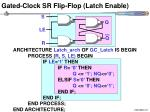Gated-Clock SR Flip-Flop (Latch Enable)

S

Q

LE

Q

R

ARCHITECTURE Latch_arch OF GC_Latch IS BEGINPROCESS (R, S, LE) BEGIN IF LE=‘1’ THENIF R= ‘0’ THEN Q <= ‘1’; NQ<=‘0’;ELSIF S=‘0’ THEN Q <= ‘0’; NQ<=‘1’;END IF; END IF; END PROCESS;END ARCHITECTURE;

CWRU EECS 317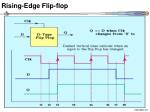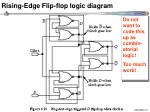Rising-Edge Flip-flop logic diagram

Do not want to code this up as combin-atorial logic!

Too much work!

CWRU EECS 317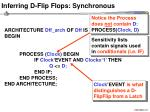Inferring D-Flip Flops: Synchronous

Notice the Process does not contain D:PROCESS(Clock, D)

Clock’EVENT is what distinguishes a D-FlipFlip from a Latch

ARCHITECTURE Dff_arch OF Dff ISBEGIN

PROCESS (Clock)BEGIN IF Clock’EVENT AND Clock=‘1’ THEN Q <= D; END IF; END PROCESS;END ARCHITECTURE;

Sensitivity lists contain signals used in conditionals (i.e. IF)

CWRU EECS 317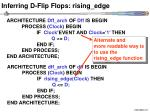Inferring D-Flip Flops: rising_edge

Alternate andmore readable way is to use the rising_edge function

ARCHITECTURE Dff_arch OF Dff IS BEGINPROCESS (Clock)BEGIN IF Clock’EVENT AND Clock=‘1’ THEN Q <= D; END IF; END PROCESS;END ARCHITECTURE;

ARCHITECTURE dff_arch OF dff IS BEGINPROCESS (Clock)BEGIN IF rising_edge(Clock) THEN Q <= D; END IF; END PROCESS;END ARCHITECTURE;

CWRU EECS 317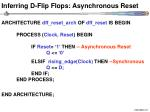Inferring D-Flip Flops: Asynchronous Reset

ARCHITECTURE dff_reset_arch OF dff_reset IS BEGINPROCESS (Clock, Reset)BEGIN IF Reset= ‘1’ THEN -- Asynchronous ResetQ <= ‘0’

ELSIF rising_edge(Clock) THEN--Synchronous Q <= D; END IF; END PROCESS;

END ARCHITECTURE;

CWRU EECS 317Inferring D-Flip Flops: Synchronous Reset

PROCESS (Clock, Reset)BEGIN IF rising_edge(Clock) THEN IF Reset=‘1’ THENQ <= ‘0’ ELSE Q <= D; END IF; END IF;END PROCESS;

Synchronous Reset

Synchronous FF

PROCESS (Clock, Reset)BEGIN IF Reset=‘1’ THENQ <= ‘0’ ELSIF rising_edge(Clock) THEN Q <= D; END IF;END PROCESS;

Asynchronous Reset

Synchronous FF

CWRU EECS 317D-Flip Flops: Asynchronous Reset & Preset

PROCESS (Clock, Reset, Preset)BEGIN IF Reset=‘1’ THEN --highest priorityQ <= ‘0’; ELSIF Preset=‘1’ THENQ <= ‘0’;ELSIF rising_edge(Clock) THEN Q <= D; END IF;

END PROCESS;

CWRU EECS 317VHDL clock behavioral component

ENTITY clock_driver IS

GENERIC (Speed: TIME := 5 ns);

PORT (Clk: OUT std_logic);END;

ARCHITECTURE clock_driver_arch OF clock_driver IS

SIGNAL Clock: std_logic := ‘0’;

BEGIN

Clk <= Clk XOR ‘1’ after Speed;

Clock <= Clk;

END ARCHITECTURE;CONFIGURATION clock_driver_cfg OF clock_driver ISFORclock_driver_arch END FOR;END CONFIGURATION;

CWRU EECS 317FSM Representations

CWRU EECS 317Moore Machines

CWRU EECS 317Simple Design Example

ENTITY FSM_Parity IS

PORT (i1: IN std_logic;

o1: OUT std_logic;

CLK: IN std_logic; --Clock

RST: IN std_logic --Reset

); END;

CWRU EECS 317State Encoding

– –State Encoding is sequentially done by VHDL

TYPE FSMStates IS (s1, s2); --s1=0, s2=1

SIGNAL State, NextState: FSMStates;

– –The non-sequential case requires the following

ATTRIBUTE FSMencode: string;

ATTRIBUTE FSMencode of FSMStates: TYPE IS “1 0”;

CWRU EECS 317Simple Design Example

PROCESS (State, i1) BEGIN

CASE State IS

WHEN s1 => if i1=‘1’ then NextState <= s2;

else NextState <= s1; end if;

WHEN s2 => if i1=‘1’ then NextState <= s1;

else NextState <= s2; end if;

WHEN OTHERS => NextState <= NextState;

END CASE;

END PROCESS;

CWRU EECS 317FSM Controller: Current State Process

ARCHITECTURE FSM_Parity_arch OF FSM_Parity IS

TYPE FSMStates IS (s1, s2);

SIGNAL State, NextState: FSMStates;

BEGIN

PROCESS (State, i1) BEGIN

CASE State IS

WHEN s1 => if i1=‘1’ then NextState <= s2;

else NextState <= s1; end if;

WHEN s2 => if i1=‘1’ then NextState <= s1;

else NextState <= s2; end if;

WHEN OTHERS => NextState <= NextState;

END CASE;

END PROCESS;

WITH State SELECT

o1 <= ‘0’ WHEN s1,

‘1’ WHEN s2,

‘1’ WHEN OTHERS; - - X, L, W, H, U

CWRU EECS 317Alternative: less coding

ARCHITECTURE FSM_Parity_arch OF FSM_Parity IS

TYPE FSMStates IS (s1, s2);

SIGNAL State, NextState: FSMStates;

BEGIN

PROCESS (State, i1) BEGIN

CASE State IS

WHEN s1 => if i1=‘1’ then NextState <= s2;

else NextState <= s1;

end if;

o1 <= ‘0’;

WHEN s2 => if i1=‘1’ then NextState <= s1;

else NextState <= s2;

end if;

o1 <= ‘1’;

WHEN OTHERS =>

o1 <= ‘1’; NextState <= NextState;

END CASE;

END PROCESS;

Important Note: every input to the state machine must be in the PROCESS sensitivity list

Important Note: every WHEN must assign the same set of signals: i.e. NextState and o1. if you miss one assignment latches will show up!

CWRU EECS 317FSM controller: NextState Process

PROCESS (CLK, RST) BEGIN

IF RST='1' THEN -- Asynchronous Reset

State <= s1;

ELSIF rising_edge(CLK) THEN

State <= NextState;

END IF;

END PROCESS;

END ARCHITECTURE;

CONFIGURATION FSM_Parity_cfg OF FSM_Parity IS

FOR FSM_Parity_arch

END FOR;

END CONFIGURATION;

CWRU EECS 317Logic Implementations

Synthesis

CWRU EECS 317Assignment #6

a) Write the VHDL synchronous code (no latches!) and test bench for the coke II machine. Note: the dc_shell synthesis analyze command will tell you if you inferred latches. Hand code and simulation using the Unix script command.

b) Synthesize the your design and hand in the logic diagram, Unix script include cell, area, timing report.

CWRU EECS 317